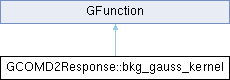GammaLib  1.7.0.dev
GCOMD2Response::bkg_gauss_kernel Class Reference
Inheritance diagram for GCOMD2Response::bkg_gauss_kernel:## Public Member Functions

bkg_gauss_kernel (const double &ereco, const double &e0, const double &sigma)

double eval (const double &e)
Computes Compton background multiplied with Gaussian. More...Public Member Functions inherited from GFunction
GFunction (void)
Void constructor. More...

GFunction (const GFunction &function)
Copy constructor. More...

virtual ~GFunction (void)
Destructor. More...

GFunctionoperator= (const GFunction &function)
Assignment operator. More...

## Protected Attributes

double m_ereco

double m_e0
Incident energy (MeV) More...

double m_wgt
Inverse of Gaussian standard deviation (1/MeV) More...Protected Member Functions inherited from GFunction
void init_members (void)
Initialise class members. More...

void copy_members (const GFunction &function)
Copy class members. More...

void free_members (void)
Delete class members. More...

## Detailed Description

Definition at line 113 of file GCOMD2Response.hpp.

## Constructor & Destructor Documentation

 GCOMD2Response::bkg_gauss_kernel::bkg_gauss_kernel ( const double & ereco, const double & e0, const double & sigma )
inline

Definition at line 115 of file GCOMD2Response.hpp.

## Member Function Documentation

 double GCOMD2Response::bkg_gauss_kernel::eval ( const double & e )
virtual

Computes Compton background multiplied with Gaussian.

Parameters
 [in] e Energy (MeV).
Returns
Compton background multiplied with Gaussian

Computes the Compton background multiplied with a Gaussian using

$B_{\rm c}(E|E',E_0) = \theta(E_0-E) \exp \left( -\frac{1}{2} \frac{(E-E')^2}{\sigma^2(E')} \right)$

where $$\theta(x)$$ is a step function that is 1 for positive $$x$$ and 0 for non-positive $$x$$, $$E_0$$ is the position of the photo peak, $$E'$$ is the reconstructed energy, $$\sigma(E')$$ is the standard deviation at the reconstructed energy, and $$E$$ is the energy over which the convolution is performed.

Implements GFunction.

Definition at line 986 of file GCOMD2Response.cpp.

References exp().

## Member Data Documentation

 double GCOMD2Response::bkg_gauss_kernel::m_e0
protected

Incident energy (MeV)

Definition at line 124 of file GCOMD2Response.hpp.

 double GCOMD2Response::bkg_gauss_kernel::m_ereco
protected

Definition at line 123 of file GCOMD2Response.hpp.

 double GCOMD2Response::bkg_gauss_kernel::m_wgt
protected

Inverse of Gaussian standard deviation (1/MeV)

Definition at line 125 of file GCOMD2Response.hpp.

The documentation for this class was generated from the following files: